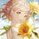# Standard Deviation - Sum Of The Squares Minus Square Of The Sums

צפיות ‎789‎
789
Introduction

The standard deviation measure the dispersion of a data set, in short this metric will tell you if your data is on average closer or farther away from the mean. Its one of the most important tools in statistics and living without it is pretty much impossible, without it you can forget about Bollinger-bands, CCI , and even the LSMA (ouch this hurt).

Now i don't want to extend myself about the standard deviation since that would require a huge post but i want to show you how to calculate the standard deviation from the stdev pinescript function.

Sum Of The Squares Minus Square Of The Sums

Any metric calculated from a moving average can be classified as "running", this mean that the metric constantly update itself and is not constant, this is why it is better to say "running standard deviation" but its okay. If we use the standard calculation for the standard deviation which would be sqrt( sma (pow(close - sma ,2))) we might get something totally different from the stdev function :

In white the pine stdev function and in red the standard calculation of both period 4, its clear that both are not the same, one might try to use the Bessel's correction but that won't do either, this is because most technical analysis tools will calculate the square root of the "Sum Of The Squares Minus Square Of The Sums" method to estimate the standard deviation

Another way is to use :

a = sqrt( sma (pow(close,2),length) - pow( sma (close,length),2))

By returning the difference we might still see some errors :

Nothing relevant of course.

Conclusion

Some of you might already be aware of this but a reminder is always good since it can be confusing to make what can be considered the good standard deviation formula and then have something totally different from the pine function, i hope this post will be useful and that you learned something from it.

You can check my indicator papers here : https://figshare.com/authors/Alex_Pierrefeu/7339466Hi, sorry to be a Luddite... but are you saying this is a more accurate standard deviation? also to do a standard deviation of a Moving average and the price, where would i put the moving average in the calculation.

Thanks

H
השבDannyBaker
@DannyBaker, The method i showcased should be the one used to calculate the stdev function in pine thats all. Its a biased estimate and it might be useful to know how its made.
השבalexgrover
@alexgrover, Okay thanks, that will be useful, and where should i put my indicator in the formula against the price
השבDannyBaker
@DannyBaker, I'am afraid i don't fully understand. You can calculate the standard deviation of "y" by replacing "close" in the code by "y" but you should still use the stdev function since its faster.
השבalexgrover
@alexgrover, i have this as a standard deviation calculation or price against a moving average
// z = 0.0
// for i = 0 to cycleLen1 - 1
// z := z + (abs(hlc3 - ma))*(abs(hlc3 - ma))
// dev = sqrt(z / cycleLen1)
השב